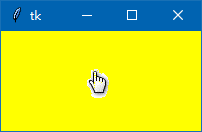# tkinter窗体属性赋值及获取窗体属性值的方法

### 了解窗体有什么属性

```from tkinter import *
root=Tk()
print(root.keys())   # 输出窗体的属性
root.mainloop()```

['bd', 'borderwidth', 'class', 'menu', 'relief', 'screen', 'use', 'background', 'bg', 'colormap', 'container', 'cursor', 'height', 'highlightbackground', 'highlightcolor', 'highlightthickness', 'padx', 'pady', 'takefocus', 'visual', 'width']

看上去窗体有很多属性，但是，我们平时常用的属性值就那么几个，其它不太常用的属性设置，我们可以暂时不用管它们。

### 用config()方法设置窗体属性

```root.config(bg='yellow',cursor='hand2',width=200,height=100)
root.configure(bg='yellow',cursor='hand2',width=200,height=100)```

```from tkinter import *
root=Tk()
root.config(bg='yellow',cursor='hand2',width=200,height=100)
root.mainloop()```### 通过字典键设置窗体属性

```root['bg']='yellow'
root['cursor']='hand2'
root['width']=200
root['height']=100```

这种方法，如果为一个不存在的属性设置，就会被 系统报TclError 错误。

### 获取窗体属性之方法１

```root['height']    # 获取窗体高度
root['bg']      # 获取窗体背景颜色
root['cursor']   # 获取窗体的鼠标样式```

我们可以把获取的属性性赋值给别的变量，或者直接打印出来。

```from tkinter import *
root=Tk()
root['height']=300
root['bg']='red'
root['cursor']='hand2'
print(root['height'])
print(root['bg'])
print(root['cursor'])
root.mainloop()```

上面代码中，我们可以用print把窗体的属性值打印出来。

### 获取窗体属性值之方法２

```root.cget('height')
root.cget('bg')
root.cget('cursor')```

```from tkinter import *
root=Tk()
root.config(height=100,bg='red',cursor='hand2')
print(root.cget('height'))
print(root.cget('bg'))
print(root.cget('cursor'))
root.mainloop()```

### 其它方法获取窗体的宽和高

root.winfo_width()  # 获取窗体的宽

root.winfo_height()  # 获取窗体的高

```from tkinter import *
root=Tk()
root.geometry('300x150+888+444') # 设置窗体大小和位置
root.update()  # 刷新窗体
print(root.winfo_width())  # 输出窗体宽
print(root.winfo_height()) # 输出窗体高
root.mainloop()```

### 其它方法获取窗体的位置

root.winfo_x()  # 获取窗体左边距

root.winfo_y()  # 获取窗体上边距

```from tkinter import *
root=Tk()
root.geometry('300x150+888+444') # 设置窗体大小和位置
root.update()  # 刷新窗体
print(root.winfo_x())  # 输出窗体左边距
print(root.winfo_y()) # 输出窗体上边距
root.mainloop()```

### tkinter窗体居中显示的代码

root.geometry('±100±200'

-数值，表示窗口右边离屏幕右边的距离

-数值，表示窗口下边离屏幕下边的距离

```root.geometry('+100+200')  # 窗体在屏幕左上角
root.geometry('-100-200')  # 窗体在屏幕右下角
root.geometry('+100-200')  # 窗体在屏幕左下角
root.geometry('-100+200')  # 窗体在屏幕右上角```

```a,b=300,150       # a为窗体宽度，b为窗体的高度
c=(root.winfo_screenwidth()-a)/2    # (屏幕宽度-窗体宽度)/2
d=(root.winfo_screenheight()-b)/2   # (屏幕高度-窗体高度)/2
root.geometry('%dx%d+%d+%d' % (a,b,c,d))　# c为窗口左边离屏幕左边的距离，d为窗口上边离屏幕上边的距离```

root.geometry('%dx%d+%d+%d' % (a,b,c,d)) 这一代码需要你懂得python的字符串格式化知识，这里是使用%操作符来格式化字符串。

root.winfo_screenwidth() 为获取屏幕的宽度，括号不能省

root.winfo_screenheight()  为获取屏幕的高度，括号不能省。

<< 上一篇 下一篇 >>